# Java Program Structure and Data Types

This lesson will teach you the structure of a Java program. How to set the path, how to compile, and how to finally run your program? Moreover it will introduce data types.

## Java Sample Program

Here is a sample program that has been written in Java language. This example is just to give you an idea that how can one write code for Java.

```    public class Omer_Class
{
public static void main(String arg[ ] )
{
System.out.println("Sample work at omerjaved.com");
}
}
```

Don’t be confused at this point. This program is just to show you the structure of a Java Program. I will discuss its detail in the coming lesson(s). This program prints "Sample work at omerjaved.com" on the output.

The code in TextPad will look like :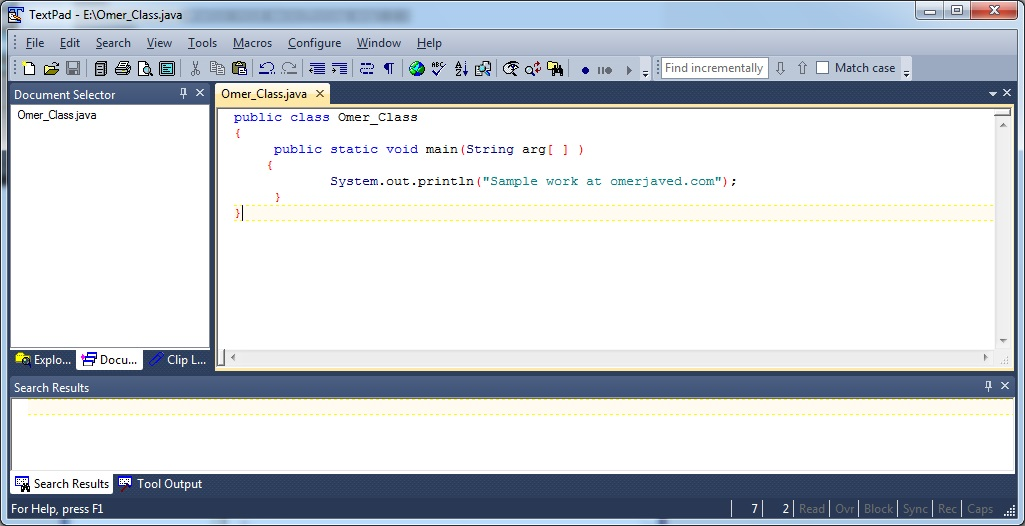While the screen of NotePad is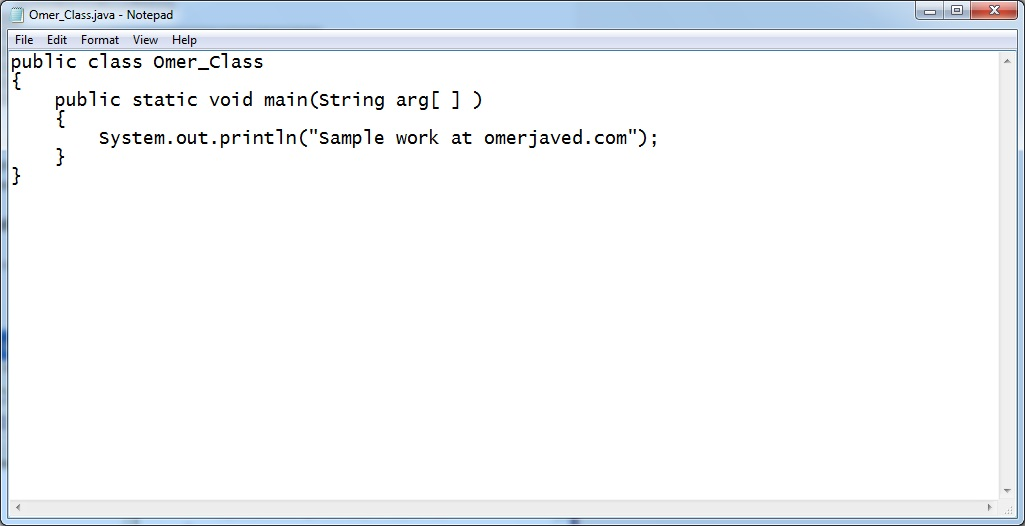Note that TextPad provides more functionalities than NotePad like compiling and running your program while in case of NotePad you have to use DOS Prompt for this purpose.

### Is Any Path Setting Required?

Yes, if you compile your java program on DOS Prompt then path setting is essentially required. For example, "set path=e:/jdk1.x/bin" is the command to set the path where x is the version for example 1.4, 1.7 etc. Note that bin in the above path is the folder name where javac.exe exists.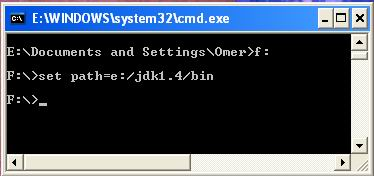Note that if you are using TextPad then there is no need of such path setting as they are already set.

## How to Compile Java Program?

To compile the Java file javac.exe is used. If you are compiling on DOS Prompt then the command is javac Omer_Class.java where Omer_Class.java is the java file to be compiled.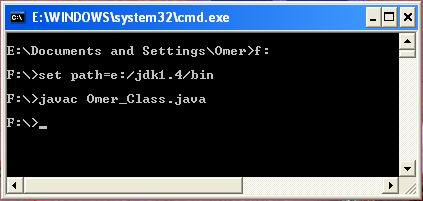While in TextPad case you have to press only “Ctrl+1” key to compile. Note that after compilation a new file would be created with “.class” extension i.e. “Omer_Class.class”

## How To Run Java Program?

After the creation of “.class” file, its time to run it. For this purpose java.exe file is used. If you are using DOS Prompt to run then the command would be as following:
java Omer_Class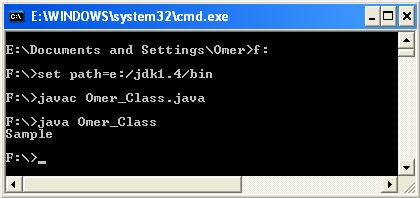In case, if you are working on TextPad then you would need to do only one thing and that is the pressing of “Ctrl+2” key.

## How To Give Comment(s) In Java

Notice that you can give single-line or multi-line comments by using // and /*text*/ respectively. Comments are just to remember something about particular method etc. and they are actually ignored by compiler. Programmers use comments for proper documentation. For example,

```    // Single line comment

/*
Multi – line comment
*/
```

## Java Data Types

The data used in a programming language like Java, has particular type. e.g. numeric numbers can be int (means integer), float (means real number as you see in Mathematics) etc. There are 8 data types in Java depending on the data used i.e. byte, short, int, long, float, double, char, and boolean.

The first four data types lie in integer data type category. Next two lie in floating point data type.
char is to store a character. For example,

```    char ch ;
ch = ‘x’;
```

while boolean is used for condition purpose. Its value can be either true or false. For example, boolean flag = true;

### Difference Between Integer and Floating Point Data Types

The integer data types are used to store whole numbers like 32, -456, 7000 etc. while floating point data type is use to store decimal numbers like 3.6, -2.6E+7 (means –2.6 raise to the power 7) etc.

### Incorrect Data

You have to assign data (value) to a variable according to the variable’s data type. For example,

```	int  a;
a = 1.3E+01;
```

This is not correct because integer variable ‘a’ cannot have such format.
Similarly spaces are not allowed within a number e.g. int a = 56 8; would be incorrect integer number.

3 out of 5 based on 11 user ratings